# 知识图谱问答系统补课——NPL

origin from :datawhale team

Word2Vec

Word vectors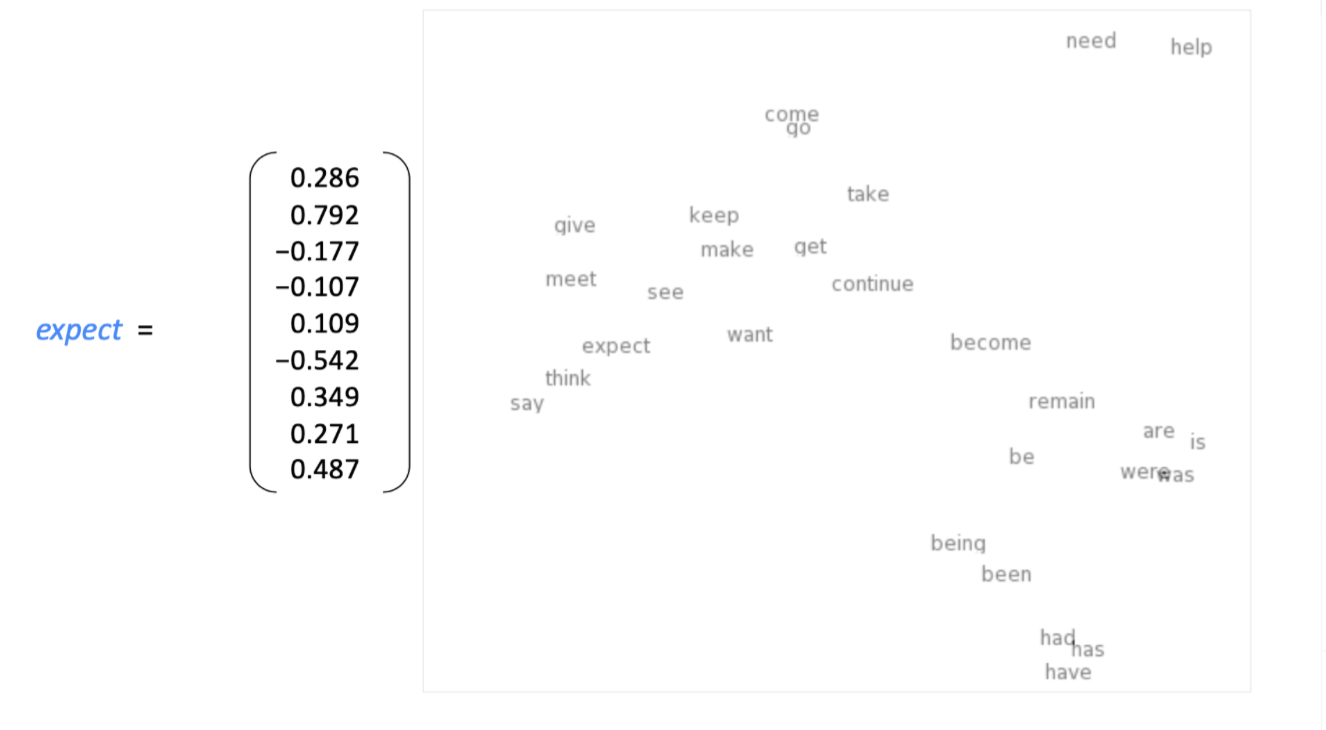Word2vec：Overview

Word2vec(Mikolov et al. 2013) 是一种学习词向量的框架，包含大量的文本语料，固定词表中的每一个单词由一个词向量表示，文本中的每个单词位置 t，有一个中心词c，和它的上下文 o（除了 c 的外部单词）。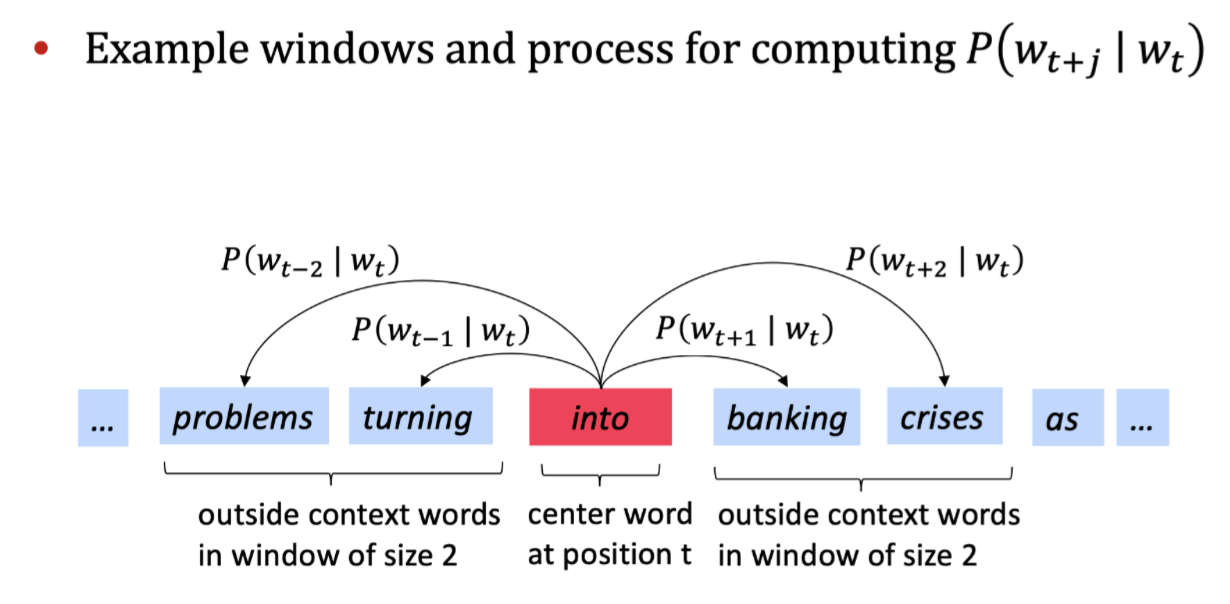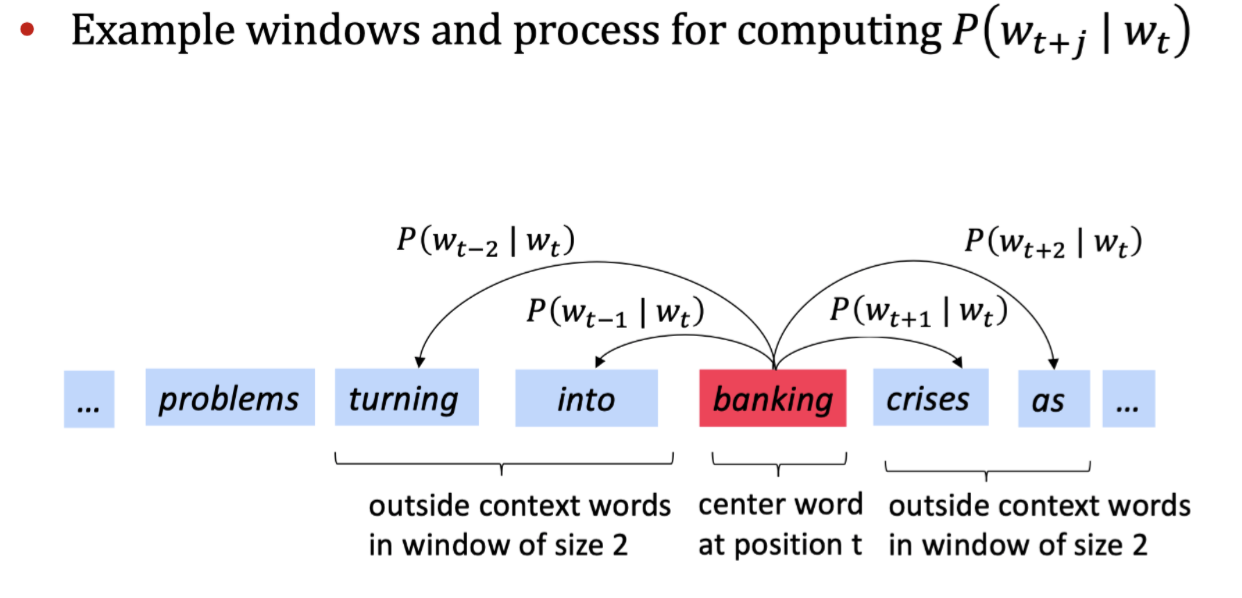Word2vec的目标函数：

l
i
k
e
l
i
h
o
o
d
=
L
(
θ
)
=

t
=
1
T

m

j

m

j

0
p
(
w
t
+
j

w
t
;
θ
)
likelihood=L( heta)=prod_{t=1}^{T}prod_{-m le j le m\ j e0}^{}p(w_{t+j}|w_t; heta)
likelihood=L(θ)=t=1∏T​−m≤j≤m    j​=0∏​p(wt+j​∣wt​;θ) 注：
θ
heta
θ 是需要优化的参数

J

θ

=

1
T
l
o
g
L
(
θ
)
=

1
T

t
=
1
T

m

j

m

j

0
l
o
g
P
(
w
t
+
j

w
t
;
θ
)
J（ heta）=-frac 1TlogL( heta)=-frac1Tsum_{t=1}^Tsum_{-mle jle m \ j e 0}logP(w_{t+j}|w_t; heta)
J（θ）=−T1​logL(θ)=−T1​t=1∑T​−m≤j≤m    j​=0∑​logP(wt+j​∣wt​;θ) ） 注：

J
(
θ
)
J( heta)
J(θ)为损失函数（这里是平均负对数似然）；

log函数方便将乘法转化为求和（优化处理）

P
(
w
t
+
j

w
t
;
θ
)
P(w_{t+j}|w_t; heta)
P(wt+j​∣wt​;θ)?

v
w
v_w
vw​ 和
u
w
u_w
uw​

v
w
v_w
vw​ ：当 w 是中心词时

u
w
u_w
uw​ ：当 w 是上下文单词时

P
(
o

c
)
=
e
x
p
(
u
o
T
v
c
)

w
ϵ
V
e
x
p
(
u
w
T
v
c
)
P(o|c)=frac {exp(u_o^Tv_c)}{sum_{wepsilon V}exp(u_w^Tv_c)}
P(o∣c)=∑wϵV​exp(uwT​vc​)exp(uoT​vc​)​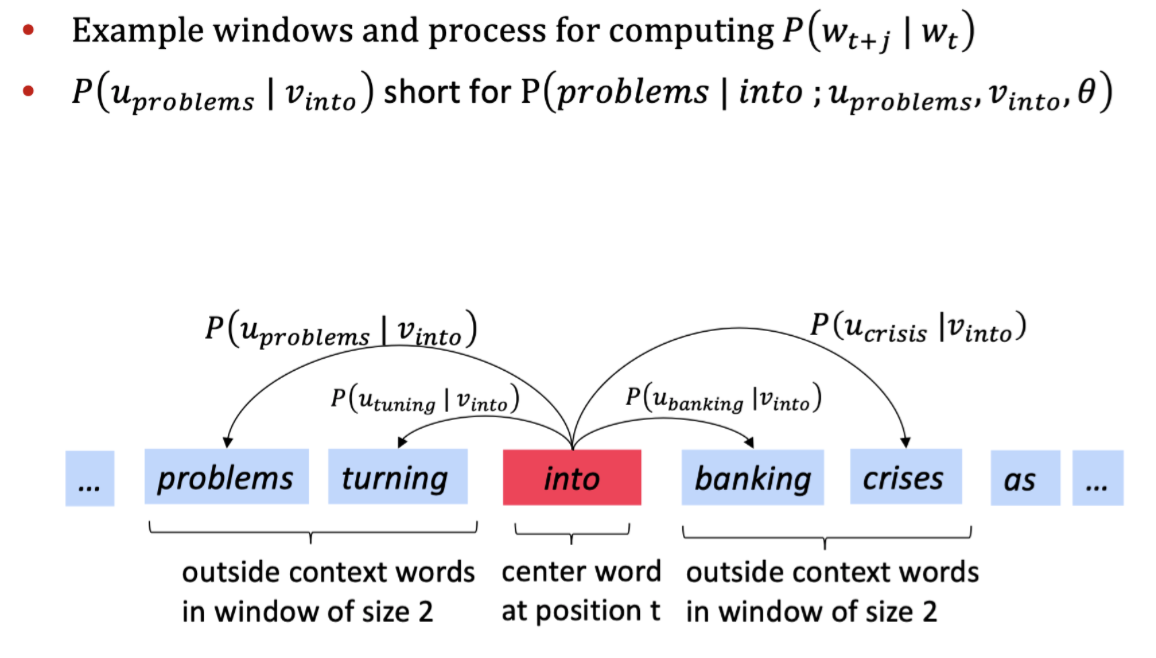在概率函数中：
P
(
o

c
)
=
e
x
p
(
u
o
T
v
c
)

w
ϵ
V
e
x
p
(
u
w
T
v
c
)
P(o|c)=frac {exp(u_o^Tv_c)}{sum_{wepsilon V}exp(u_w^Tv_c)}
P(o∣c)=∑wϵV​exp(uwT​vc​)exp(uoT​vc​)​ 分子取幂函数使得始终可以为正

u
o
u_o
uo​ 和向量
v
c
v_c
vc​ 点乘，点乘结果越大，向量之间越相似

u
T
v
=
u

v
=

i
=
1
n
u
i
v
i
u^Tv=u·v=sum_{i=1}^nu_iv_i
uTv=u⋅v=∑i=1n​ui​vi​

softmax函数进行归一化（深度学习中常用）：
R
n

R
n
Bbb{R^n} o Bbb{R^n}
Rn→Rn

（公式
x
s
o
f
t
m
a
x

x

=
e
x
p
(
x
i
)

j
=
1
n
e
x
p
(
x
j
)
=
p
i
xsoftmax（x）= frac {exp(x_i)}{sum_{j=1}^nexp(x_j)}=p_i
xsoftmax（x）=∑j=1n​exp(xj​)exp(xi​)​=pi​

） 注：用于将任意值
x
i
x_i
xi​ 映射到概率分布
p
i
p_i
pi​

Word2vec objective function gradients Training a model by optimizing parameters

（通过优化参数的方式训练模型）- 最小化损失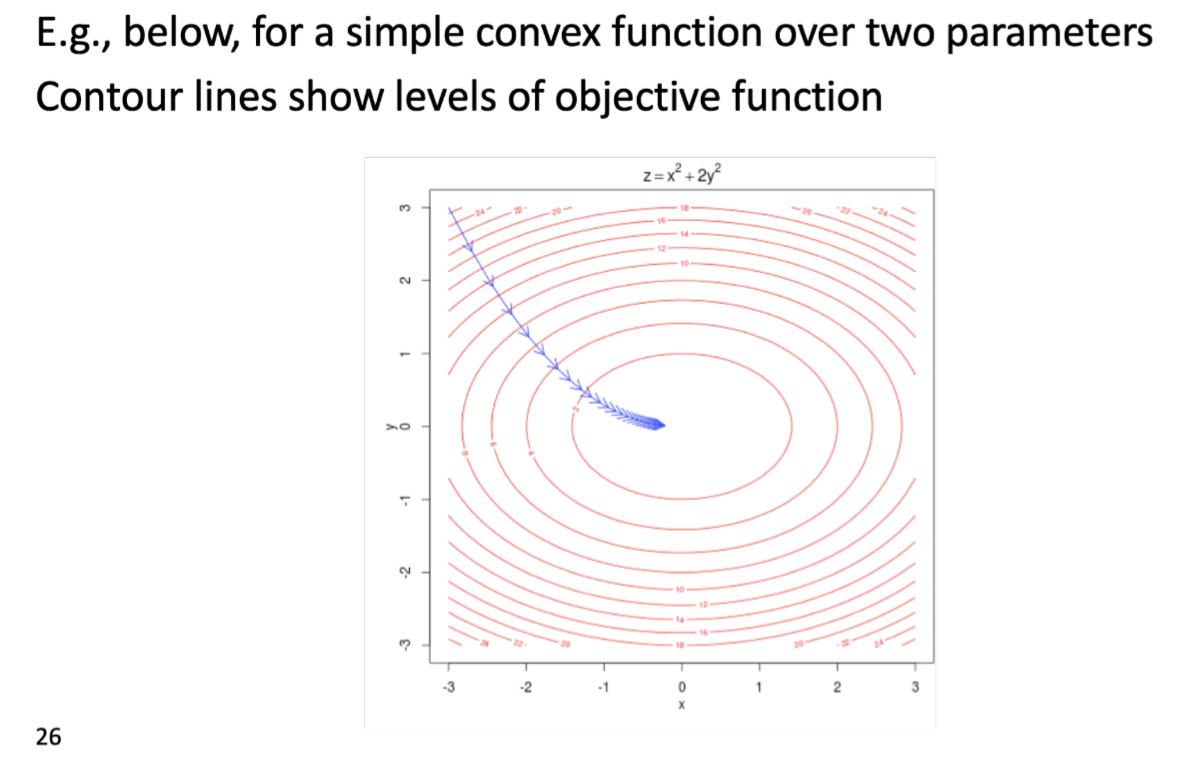To train the model: Compute all vector gradients

θ
heta
θ ,所以我们只用优化这一个参数就行。

θ
=
[
v
a
a
r
d
v
a
r
k
v
a

v
z
e
b
r
a
u
a
a
r
d
v
a
r
k
u
a

u
z
e
b
r
a
]
ϵ

R
2
d
V
heta=egin{bmatrix}v_{aardvark}\v_a\vdots\v_{zebra}\u_{aardvark}\u_a\vdots\u_{zebra} end{bmatrix}epsilon Bbb R^{2dV}
θ=⎣⎢⎢⎢⎢⎢⎢⎢⎢⎢⎢⎢⎢⎡​vaardvark​va​⋮vzebra​uaardvark​ua​⋮uzebra​​⎦⎥⎥⎥⎥⎥⎥⎥⎥⎥⎥⎥⎥⎤​ϵ R2dV 2：每个单词有两个向量

Example：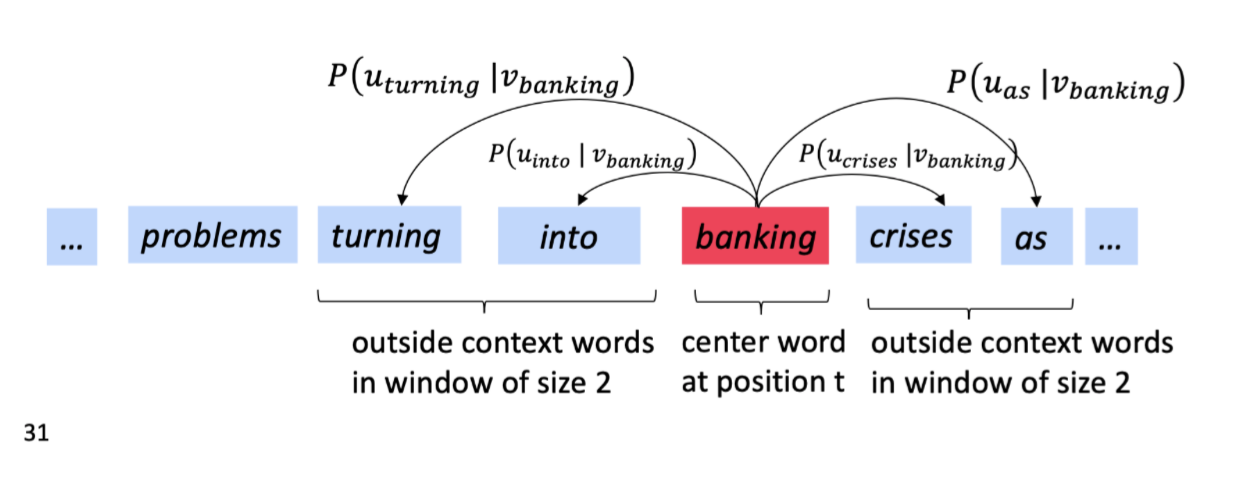5. Optimization basics

Optimization：Gradient Descent（梯度下降）

J
(
θ
)
J( heta)
J(θ) 需要最小化

θ
heta
θ ,计算
J
(
θ
)
J( heta)
J(θ) 的梯度

α
=
(
s
t
e
p

s
i
z
e
)

o
r

(
l
e
a
r
n
i
n
g

r
a
t
e
)
alpha=(step size) or (learning rate)
α=(step size) or (learning rate)
θ
n
e
w
=
θ
o
l
d

α

θ
J
(
θ
)
heta^{new}= heta^{old}-alpha abla_ heta J( heta)
θnew=θold−α∇θ​J(θ)

θ
heta
θ:
θ
j
n
e
w
=
θ
j
o
l
d

α
α
α

θ
j
o
l
d
J
(
θ
)
heta_j^{new}= heta_j^{old}-alpha frac alpha{alpha heta_j^{old}}J( heta)
θjnew​=θjold​−αα θjold​α​J(θ)

while True:
theta_grad = evaluate_gradient(J,corpus,theta)
theta = theta - alpha * theta_grad

SGD:Stochastic Gradient Descent

J
(
θ
)
J( heta)
J(θ) 是在语料文本中所有窗口的方程

while True:
window = sample_window(corpus)
theta_grad = evaluate_gradient(J,window,theta)
theta = tehta - alpha * theta_grad
# GloVe GloVe的全称是GloVe: [bal ](https://nlp.stanford.edu/projects/glove/)[Ve](https://nlp.stanford.edu/projects/glove/)[ctors for Word Representation](https://nlp.stanford.edu/projects/glove/)

GloVe目标是综合基于统计和基于预测的两种方法的优点。

Xij代表单词 i表示上下文单词

j表示在特定大小的上下文窗口（context window）内共同出现的次数。这个次数的最小单位是1，但是GloVe不这么认为：它根据两个单词在上下文窗口的距离dd.

w
i
T
w
~
j
+
b
i
+
b
~
j
=
log

(
X
i
j
)
w_{i}^{T} ilde{w}_{j}+b_{i}+ ilde{b}_{j}=log left(X_{i j} ight)
wiT​w~j​+bi​+b~j​=log(Xij​)

w
i
T
w_{i}^{T}
wiT​ 和
w
~
j
ilde{w}_{j}
w~j​是我们最终要求解的词向量；
b
i
b_{i}
bi​和
b
~
j
ilde{b}_{j}
b~j​分别是两个词向量的bias term 那它到底是怎么来的，为什么要使用这个公式？为什么要构造两个词向量
w
i
T
w_{i}^{T}
wiT​ 和
w
~
j
ilde{w}_{j}
w~j​？

J
=

i
,
j
=
1
V
f
(
X
i
j
)
(
w
i
T
w
~
j
+
b
i
+
b
~
j

log

(
X
i
j
)
)
2
J=sum_{i, j=1}^{V} fleft(X_{i j} ight)left(w_{i}^{T} ilde{w}_{j}+b_{i}+ ilde{b}_{j}-log left(X_{i j} ight) ight)^{2}
J=i,j=1∑V​f(Xij​)(wiT​w~j​+bi​+b~j​−log(Xij​))2 loss function的基本形式就是最简单的mean square loss，只不过在此基础上加了一个权重函数\$ fleft(X_{i j} ight) \$，那么这个函数起了什么作用，为什么要添加这个函数呢？我们知道在一个语料库中，肯定存在很多单词他们在一起出现的次数是很多的（frequent co-occurrences），那么我们希望：

X
i
j
X_{i j}
Xij​,那么他们应该不参与到loss function的计算当中去，也就是f(x)要满足f(x)=0

f
(
x
)
=
{
(
x
/
x
max

)
α
if
x
<
x
max
1
otherwise
f(x)=left{egin{array}{cc} left(x / x_{max } ight)^{alpha} & ext { if } x<x_{ ext {max }} \ 1 & ext { otherwise } end{array} ight.
f(x)={(x/xmax​)α1​ if x<xmax ​ otherwise ​

x
max

=
100
x_{max }=100
xmax​=100，并且发现
α
=
3
/
4
alpha=3 / 4
α=3/4时效果比较好。

BERT

## qt高速显示大图_2020海东移动密集架大图

2020海东移动密集架大图密集架想要正常的长久我的使用就必须要有密集柜的质量。而这质量的密集柜与否则包括了很多方面，包括材质，配件使用，装配等多个方面...

## python长连接框架_python之websocket【长连接的实现】

websocket技术简单来说就是实现了长连接的技术，相对于传统的ajax或者轮询，websocket主要实现了双通信，并且减少了长轮询的多次请求的时间。总的...

## app python java_APP自动化（java和python）——python操作百度浏览器

fromappiumimportwebdriverimporttimedesired_caps={'platformName':'Android','d...

## app python java_APP自动化（java和python）——java操作百度浏览器

packagejkcs;importjava.net.MalformedURLException;importjava.net.URL;importjava.util.concurrent.TimeU...